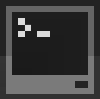ComputerCraftCurrent developersdan200, Cloudhunter
Version1.5

This tutorial will focus on the basics of Lua programs, variables, comments, functions, if statements and program structure. ComputerCraft computers are Lua interpreters, they are not simulators. In addition, Lua is a name, not an acronym. As such, it should be written "Lua", not "LUA" or "L.U.A".

## Variables

Variables are a way of storing data as aliases, much like algebra in mathematics. Variables in Lua are somewhat more intelligent than in other programming languages, such as Java. This is because they automatically detect what form they are, for example:

```variable1 = 30
variable2 = "This is a string"
variable3 = variable1
variable4 = variable2
```
• variable1 is identified as a number, because it doesn't have quotes, and because it contains only number characters.
• variable2 is identified as a string, because it is surrounded by double quotes "". Strings, unlike numbers, cannot be used in equations.
• variable3 is identified as a number (indirectly), since it equals variable1, which in itself equals 30. This ends up simplifying to "variable3 = 30".
• variable4 is identified as a string, again, because it equals variable2, as such "variable4 = variable2" becomes "variable4 = "This is a string".

These are the two types of variables, with examples of setting one variable equal to another.

## Functions

Functions allow you to place code in a separate section of the program, which won't be run with the main program, only when called. Functions are shown as:

```function name(variable1)

end
```

The word "function" defines this as a function. "name" sets what the name of the function will be, and what you type to call it. "end" shows the end of the function. Any text between "function name()" and "end" is part of the function. The brackets are used for giving data to the function. A function is called by simply typing its name, with the brackets at the end. If you are giving the function data, these go within the brackets. For example:

```--Function
function helloworld(text)
print(text)
end

--Main Program
helloworld("Hello!")
```

There were two things in that example which haven't been explained yet, as they are fairly simple.